# Transactions And Concurrency Control (Basic Level)- 1

## 10 Questions MCQ Test Question Bank for GATE Computer Science Engineering | Transactions And Concurrency Control (Basic Level)- 1

Description
This mock test of Transactions And Concurrency Control (Basic Level)- 1 for Computer Science Engineering (CSE) helps you for every Computer Science Engineering (CSE) entrance exam. This contains 10 Multiple Choice Questions for Computer Science Engineering (CSE) Transactions And Concurrency Control (Basic Level)- 1 (mcq) to study with solutions a complete question bank. The solved questions answers in this Transactions And Concurrency Control (Basic Level)- 1 quiz give you a good mix of easy questions and tough questions. Computer Science Engineering (CSE) students definitely take this Transactions And Concurrency Control (Basic Level)- 1 exercise for a better result in the exam. You can find other Transactions And Concurrency Control (Basic Level)- 1 extra questions, long questions & short questions for Computer Science Engineering (CSE) on EduRev as well by searching above.
QUESTION: 1

### The concept of locking can be used to solve the problem of 1. Lost update 2. Uncommitted dependency 3. Inconsistent data 4. Deadlock

Solution:

The concept of locking can be used to solve the problem of lost update, uncommitted dependency and prevents inconsistency among data but it is not capable of preventing the deadlock always.

QUESTION: 2

### What are the potential problems when a DBMS executes multiple transactions concurrently? 1. The lost update problem 2. The dirty read problem 3. The unrepeatable read problem 4. The phantom problem

Solution:

Lost update problem, dirty read problem , unrepeatable read problem and phantom problems are the potential problem that might occur when a DBMS executes multiple transactions concurrently.

QUESTION: 3

### A trigger is

Solution:

A trigger is actually a procedural code that is automatically executed in response to certain events on a particular table or view in a database.

QUESTION: 4

Which of the following schemes are used for ensuring atomicity?

Solution:

Following ensure atomicity:
• Log with deferred and immediate modification.

QUESTION: 5

Assume transaction A holds a shared lock R. If transaction B also requests for a shared lock on R, it will,

Solution:

Since transaction A holds only a shared lock R.
Hence any number of transaction can read the data from the requested item using shared locking. Hence B’s request will be granted immediately Hence option (b) is correct.

QUESTION: 6

Which of the following cannot be restarted?

Solution:
QUESTION: 7

Consider the following transaction schedule: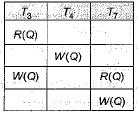The labeled precedence graph will be

Solution:

As we can see clearly T4 and T7 are followed by T3 and T4 in succeed by T3 and T7 and soon. Hence topological sequence represented by the above transactions is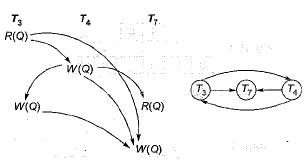QUESTION: 8

Amongst the ACID properties of a transaction, the ‘Durability' property requires that the changes made to the database by a successful transaction persist

Solution:
QUESTION: 9

Consider the following three schedules of transactions T1, T2 and T3. [Notation: In the following NYO represents the action Y(Y for read, W for write) performed by transaction Non object O.]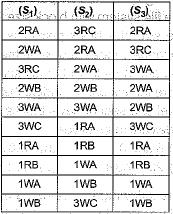Which of the following statements is TRUE?

Solution: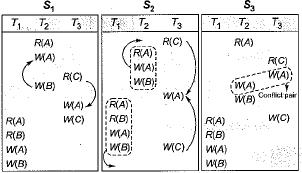As we can see S1 and S2 are conflict equivalent to each other. The equivalent serial schedule is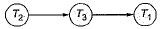While the schedule S3 is having a conflicting pair hence equivalent serial schedule is not possible. Hence option (d) is correct.

QUESTION: 10

In case Of timestamp ordering R-timestamp (Q) denotes

Solution: